## Professor Gaekwad develops a machine which  can add, subtract, combine and evaluate any two  functions. It has an input machine which can accept  the functions within its domain. It has an output  unit which displays the result and a processor used  for calculations. He gives the inputs as f(x) = cos x  and g(x) = x 2 .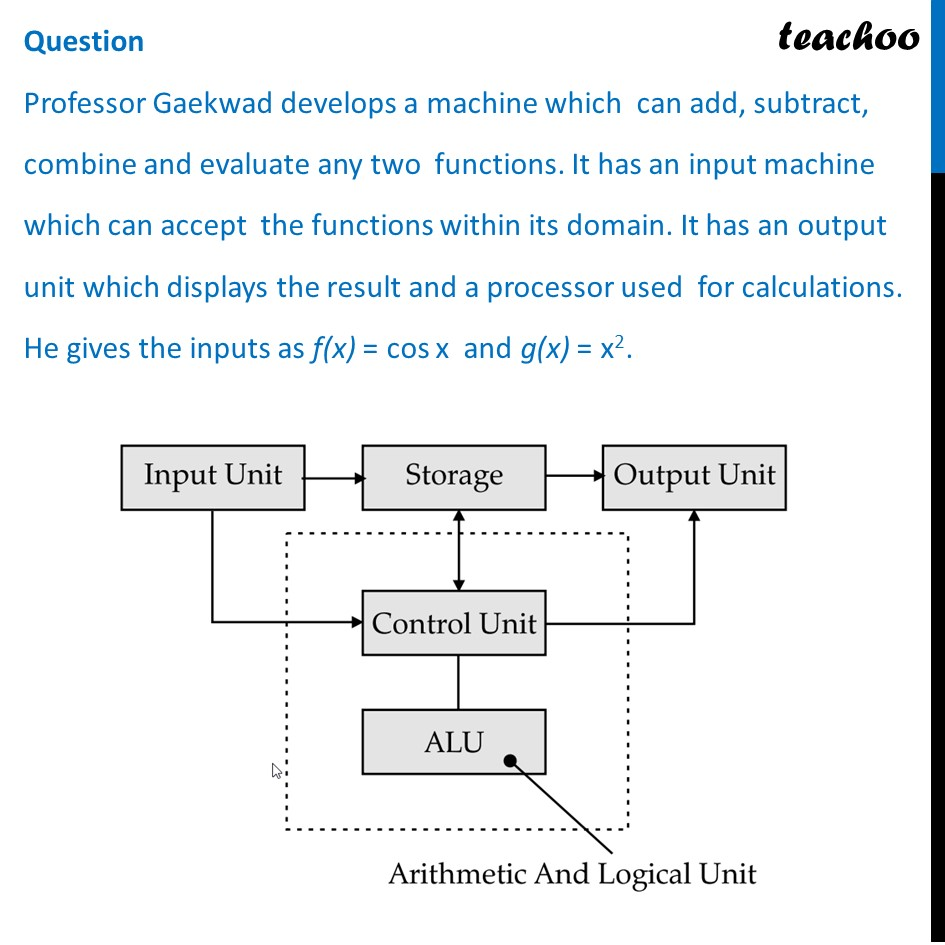## The domain of f(x) is  _______.  (a) [0, 𝜋]                 (b) [–1, 1] (c) [(-π)/2,π/2]        (d) R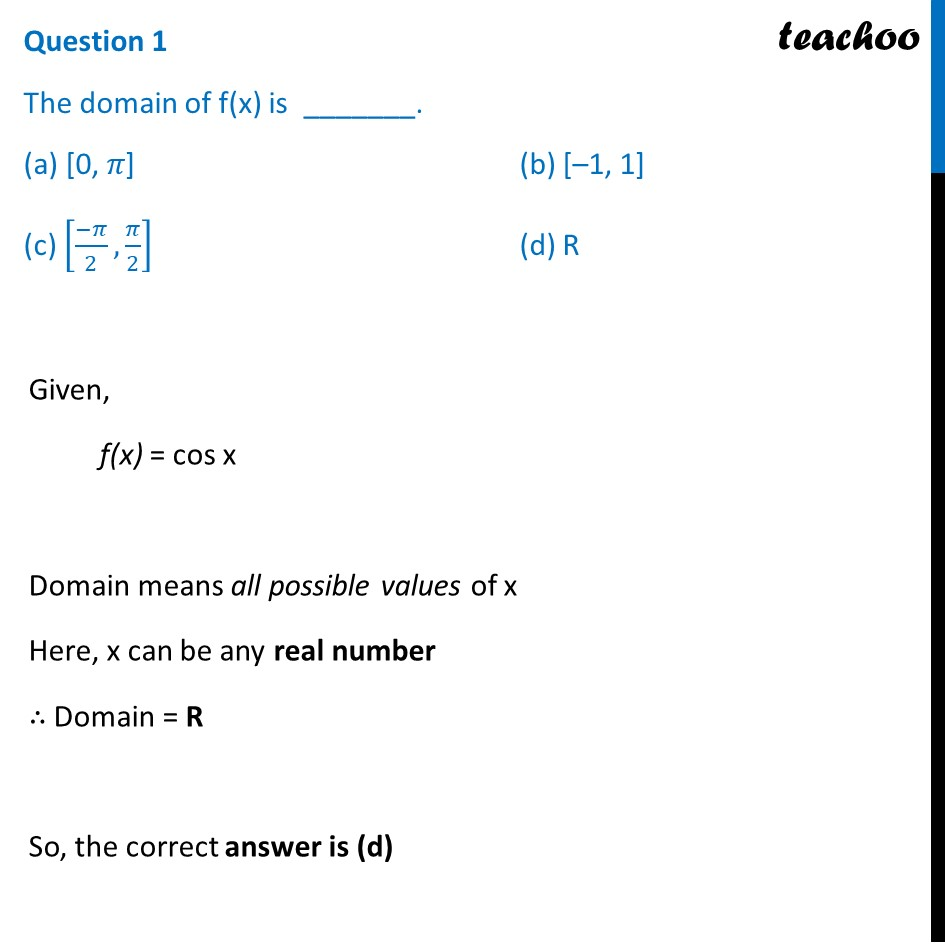## The range of g(x) is  _______.  (a) [0, 1]          (b) [–1, 1]  (c) R               (d) [0, ∞)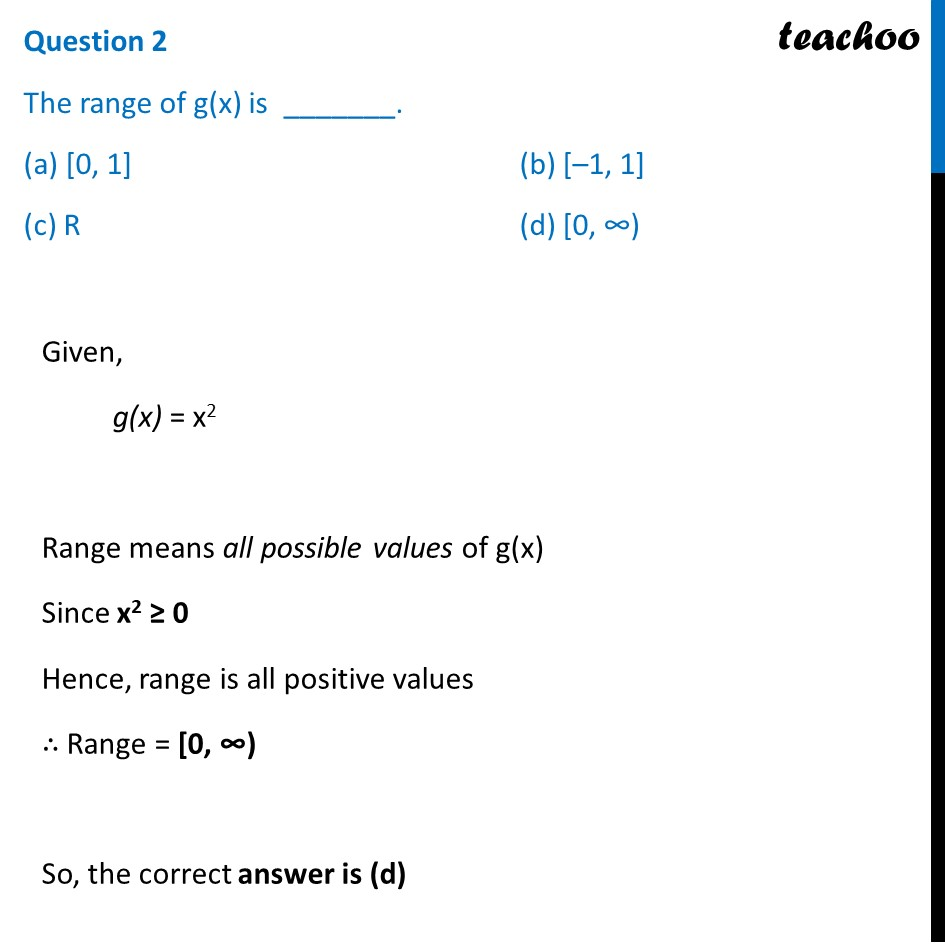## ( f + g )(0) =  _______.  (a) 0              (b) 1  (c) –1            (d) 2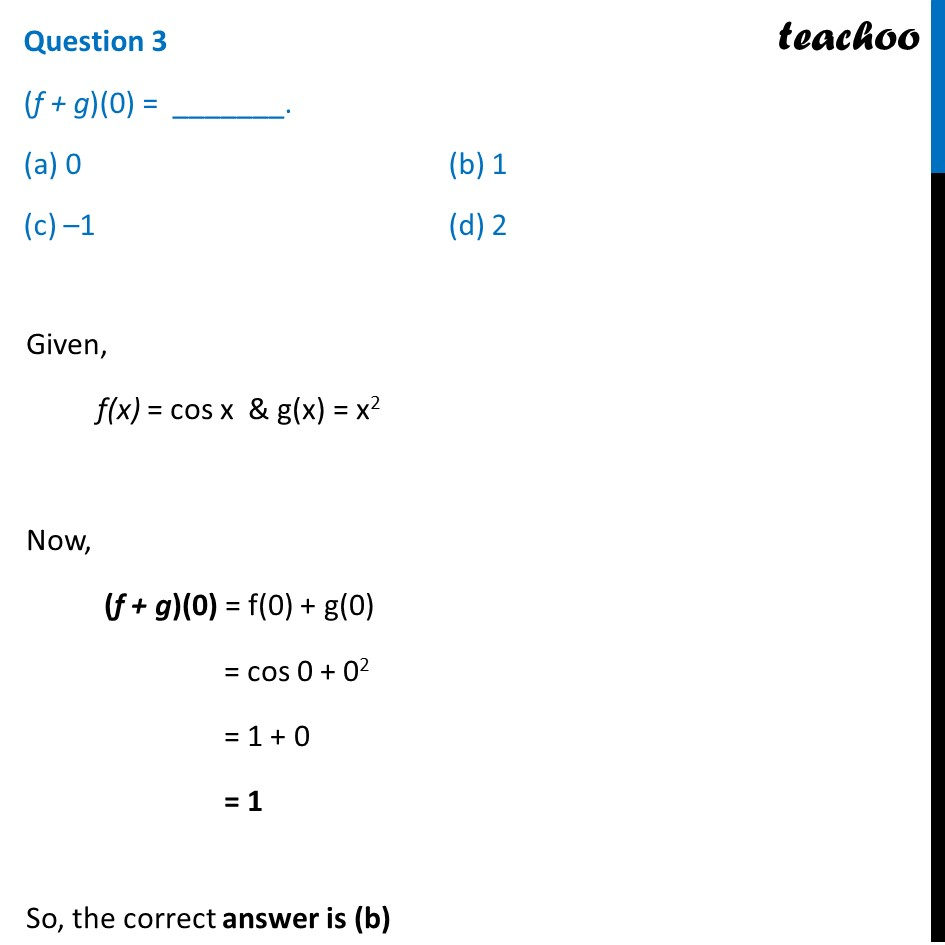## fog (x) =  _______.  (a) cos 2 x   (b) cos(x 2 )  (c) 2cos x   (d) cos 2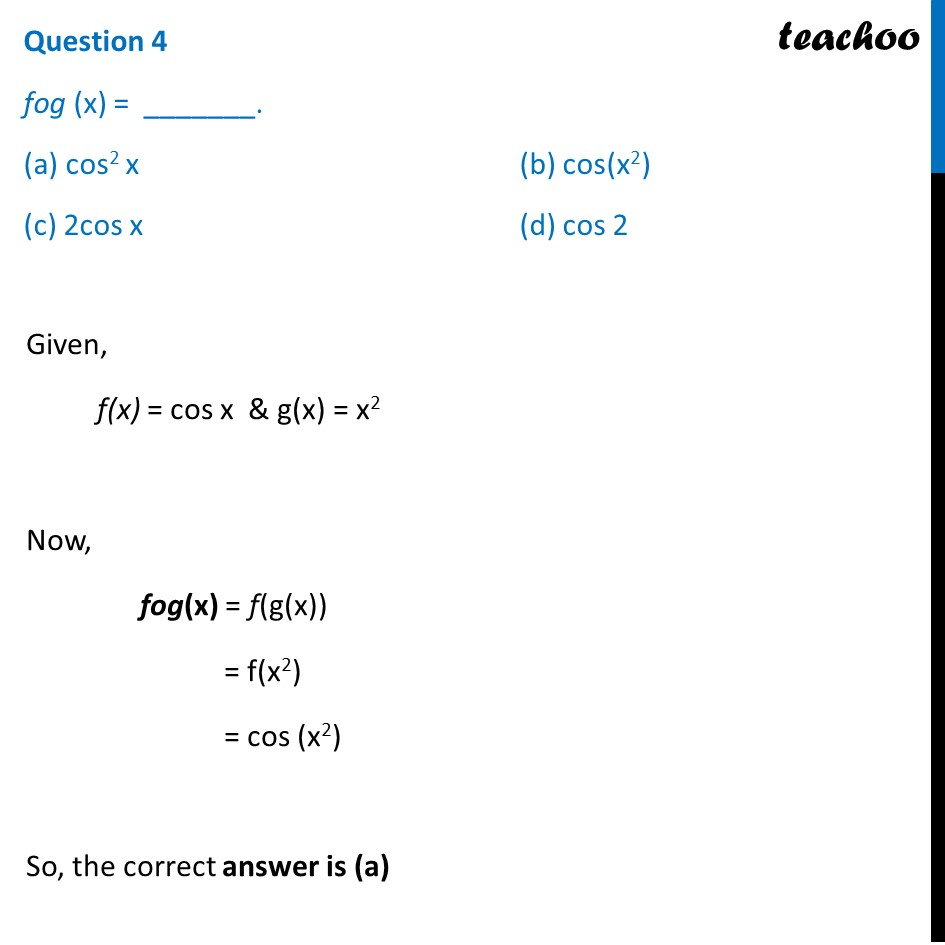## gof(x) = is  _______.  (a) cos 2 x   (b) cos(x 2 )  (c) 2cos x   (d) cos 2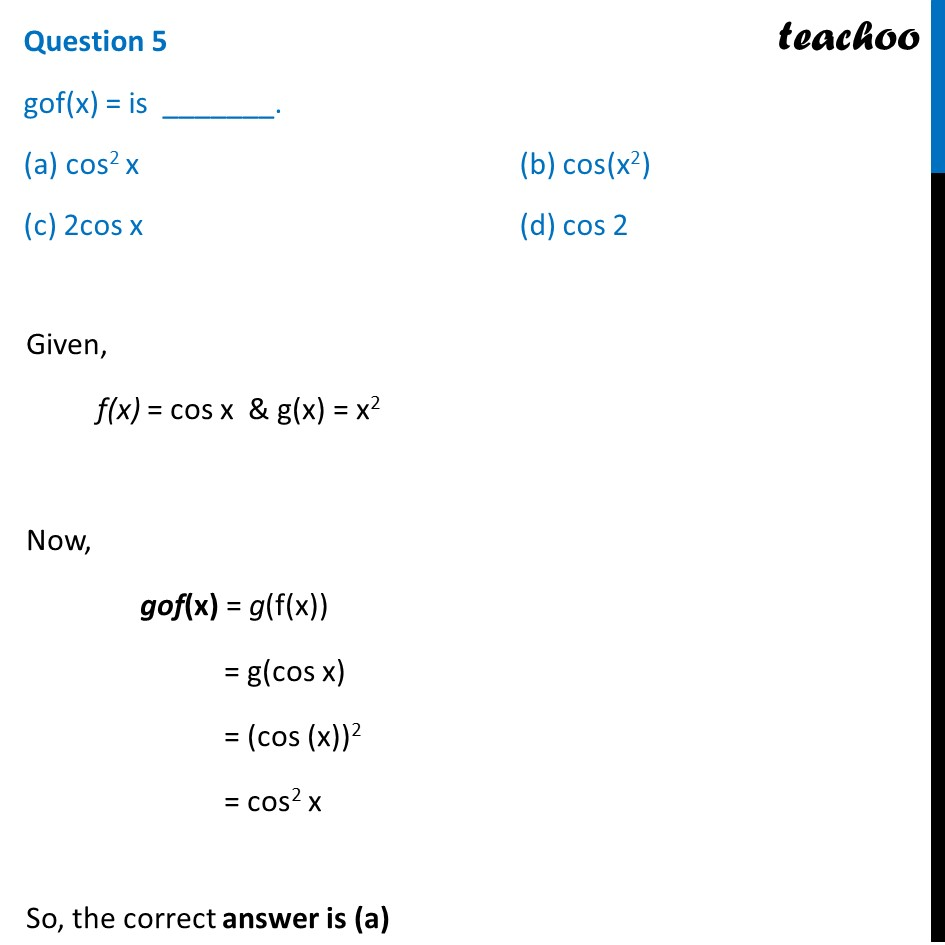1. Chapter 1 Class 12 Relation and Functions (Term 1)
2. Serial order wise
3. Case Based Questions (MCQ)

Transcript

Question Professor Gaekwad develops a machine which can add, subtract, combine and evaluate any two functions. It has an input machine which can accept the functions within its domain. It has an output unit which displays the result and a processor used for calculations. He gives the inputs as f(x) = cos x and g(x) = x2. Question 1 The domain of f(x) is _______. (a) [0, 𝜋] (b) [–1, 1] (c) [(−𝜋)/2,𝜋/2] (d) R Given, f(x) = cos x Domain means all possible values of x Here, x can be any real number ∴ Domain = R So, the correct answer is (d) Question 2 The range of g(x) is _______. (a) [0, 1] (b) [–1, 1] (c) R (d) [0, ∞) Given, g(x) = x2 Range means all possible values of g(x) Since x2 ≥ 0 Hence, range is all positive values ∴ Range = [0, ∞) So, the correct answer is (d) Question 3 (f + g)(0) = _______. (a) 0 (b) 1 (c) –1 (d) 2 Given, f(x) = cos x & g(x) = x2 Now, (f + g)(0) = f(0) + g(0) = cos 0 + 02 = 1 + 0 = 1 So, the correct answer is (b) Question 4 fog (x) = _______. (a) cos2 x (b) cos(x2) (c) 2cos x (d) cos 2 Given, f(x) = cos x & g(x) = x2 Now, fog(x) = f(g(x)) = f(x2) = cos (x2) So, the correct answer is (a) Question 5 gof(x) = is _______. (a) cos2 x (b) cos(x2) (c) 2cos x (d) cos 2 Given, f(x) = cos x & g(x) = x2 Now, gof(x) = g(f(x)) = g(cos x) = (cos (x))2 = cos2 x So, the correct answer is (a)